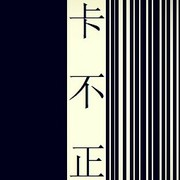[F]_ _ [G]_ _ [Em]_ _ [Am]_ _ [F]_ _ [G]_ _ [C]_ _ [C]_ _ {comment: Verse 1} 圆圈勾[F]勒成指纹 [G]　 印在[Em7]我的嘴唇 [Am]　 回忆苦[F]涩的吻痕 [G]　 是树[C]根 [C] 春去秋[F]来的茂盛 [G]　 却遮[Em7]住了黄昏 [Am]　 寒夜剩[F]我一个人 [G]　 等清[C]晨 [C] {comment: Verse 2} 世间最[F]毒的仇恨 [G]　 是有[Em7]缘却无分 [Am]　 可惜你[F]从未心疼 [G]　 我的[C]笨 [C] 荒草丛[F]生的青春 [G]　 倒也[Em7]过的安稳 [Am]　 代替你[F]陪着我的 [G]　 是年[C]轮 {start_of_chorus} 数[C]着一圈圈[F]年轮 我[G]认真 将[Em]心事都[Am]封存 密[F]密麻麻是[G]我的自[C]尊 修[C]改一次次[F]离分 我[G]承认 曾[Em]幻想过[Am]永恒 可[F]惜从没人[G]陪我演 这[C]剧本 {end_of_chorus} 可[F]惜从没人[G]陪我演 这[C]剧本 {title:年轮} {artist:张碧晨} {author:_wc_wc_}_wc_wc_